# Uncertainty principle

Heisenberg principle

One of the most important principles in quantum mechanics, which asserts that the dispersions of the values of two physical quantitiesanddescribed by non-commuting operatorsand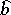with non-zero commutator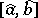in any state of a physical system cannot be simultaneously very small.

More precisely, let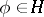,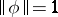, be a state of a physical system (is the Hilbert space of these states and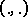is the scalar product in) and letbe the dispersion of the quantityin the state;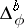is defined similarly. Then always(1)

In particular, the coordinates,,of a quantum particle and the components,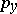,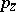of its momentum under all standard free quantizations (i.e. choices of the spaceand rules for associating self-adjoint operators acting onwith physical quantities) are represented by operators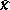,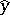,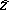and,,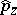such that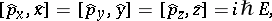whereis the identity operator onand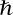is the Planck constant. Thus, for any,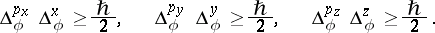(2)